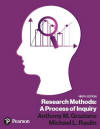﻿ Repeated Measures ANOVAGraziano & Raulin
Research Methods (9th edition)

## Comparing Two or More Dependent Samples

You learned earlier that when we are comparing two groups of participants, we must make a distinction between independent and correlated groups, because we use a different t-test for those two situations.

The t-test for correlated groups actually removes some of the variance from the denominator (see the section on comparing correlated samples). The variance that is removed is the variance that is due to individual differences, which is at least partially controlled by using a correlated groups design. You may remember that this increases our statistical power, which is our ability to detect group differences if they exist. We are going to use exactly the same strategy when comparing more than two correlated groups.

The statistical procedure for comparing two or more correlated groups is called a repeated measures ANOVA. The name comes from the fact that this procedure is most often used to analyze the results of a within-subjects design, in which all participants are measured under all conditions of the study. It can also be used to analyze data from a matched-subjects design, although this design is much less common than a within-subjects design.

Chapter 11 in the textbook describes these correlated groups designs in detail. It also describes the logic of the analysis of these designs. Let's review that logic.

With a simple one-way ANOVA, we divide the MSb by the MSw to get our F-ratio. The MSb is a function of the variability of the means, and the MSw is a function of the variability within the groups. But a good portion of the variability within groups is due to individual differences. By having the same people in each of the experimental conditions, as you do with the within-subjects design, you can control for the individual differences. This allows you in the repeated measures ANOVA to split what would normally be the MSw into two components. The subjects component is the individual differences part of the MSw. The error component is what remains after that individual differences component is removed.

This is exactly the same approach that we used with the t-test for correlated groups. We simply subtracted off the portion of the standard error that was due to individual differences. The individual differences component is often the largest part of the error term, so removing it substantially increases the size of the t in a t-test for correlated groups and the F in a repeated measures ANOVA.

## ANOVA Summary Table for a Repeated Measures ANOVA

Source

df SS MS F p
Between dfb=k-1 SSb MSb=SSb/dfb

F=MSb/MSe

Subjects dfs=N-1 SSs
Error dfe=dfbXdfs SSe

MSe=SSe/dfe

In the repeated measures ANOVA summary table, we will have an extra row, because the MSw is now divided into the MSs (mean square subjects) and the MSe (mean square error). The degrees of freedom for the between source of variance is unchanged (k-1). The degrees of freedom for the subjects term is equal to the number of participants minus 1 (N-1). Keep in mind that the participants appear in all groups in a within-subjects design. The formulas for the sums of squares are presented in the unit on computing the repeated measures ANOVA by hand. Click on the button below to view that unit.

There is also a button that will discuss how you compute a repeated measures ANOVA using computer analysis programs, although the SPSS for Windows program that can be bundled with this text (the Student Version) does not do repeated measures ANOVAs.

 Compute a Repeated Measures ANOVA  by hand Compute a Repeated Measures ANOVA using SPSS USE THE BROWSER'S BACK ARROW KEY TO RETURN

Just like in the one-way ANOVA, finding a significant F-ratio only means that at least one condition is significant different from at least one other condition. To find out which conditions are different from which other conditions requires probing techniques (either planned comparisons or post hoc tests).

The probing techniques used here are not the same as the ones used in the one-way ANOVA, because the tests here have to take into account the correlation among conditions. The procedures for planned comparisons and post hoc tests for repeated measures ANOVAs are beyond the level of this text. However, graduate level statistics texts will cover them and statistical analysis packages that perform repeated measures ANOVAS will have the options for these tests available.